Important Formulas & Examples: Trigonometric Functions - 1

# Important Formulas & Examples: Trigonometric Functions - 1 | Mathematics (Maths) Class 11 - Commerce

 Table of contentsBasic Trigonometric IdentitiesTrigonometric Functions of Allied AnglesTrigonometric Functions of Sum Or Difference of Two AnglesMultiple Angles & Sub-Multiple AnglesImportant Trigonometric RatiosConditional IdentitiesMaximum & Minimum values of Trigonometric Functions1 Crore+ students have signed up on EduRev. Have you?

Basic Trigonometric Identities

• sin² θ + cos² θ = 1 ; (-1 ≤ sin θ ≤ 1 ; -1 ≤ cos θ ≤ 1 , θ ∈ R)
• sec² θ - tan² θ = 1 ; ( |sec θ| ≥ 1, θ ∈ R)
• cosec² θ - cot² θ = 1 ; (|cosec θ| ≥ 1 , θ ∈ R)

Important Trigonometric Ratios

• sin nπ = 0 ; cos nπ = (-1)n ; tan nπ = 0 where n ∈ I
•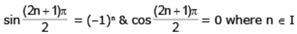Trigonometric Functions of Allied Angles

• If θ is any angle , then - θ, 90 ±θ , 180 ± θ, 270 ± θ , 360 ± θ etc. are called ALLIED ANGLES.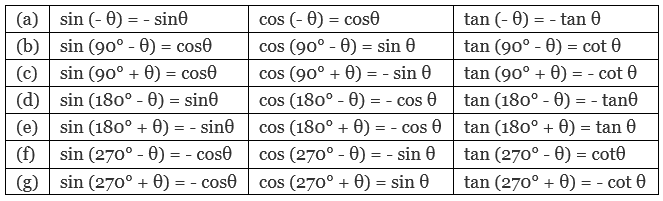Example 1. Express 1·2 radians in degree measure.

1·2 radians =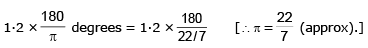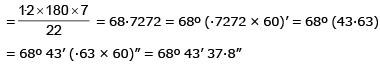Example 2. Calculate sin α if cos α = - 9/11 and α ∈ (π, 3π/2).

For any angle a belonging to the indicated interval sin a is negative, and therefore,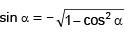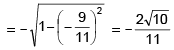Example 3. Calculate tan α if cos α = √5/5 and α ∈ (π, 3π/2)

For any angle a belonging to the indicated interval tanα is positive and cos a is negative, and therefore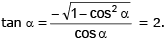Trigonometric Functions of Sum Or Difference of Two Angles

• sin (A ± B) = sinA cosB ± cosA sinB
• cos (A ± B) = cosA cosB m sinA sinB
• sin²A - sin²B = cos²B - cos²A = sin (A+B) . sin (A- B)
• cos²A - sin²B = cos²B - sin²A = cos (A+B) . cos (A - B)
•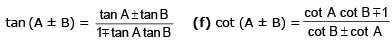➢ Factorisation of the Sum Or Difference of Two sines Or cosines:

1. sinC + sinD = 2 sin (C + D/2) cos (C - D/2)
2. sinC - sinD = 2 cos (C + D/2) sin (C - D/2)
3. cosC + cosD = 2 cos (C + D/2) cos (C - D/2)
4. cosC - cosD = - 2 sin (C + D/2) sin (C - D/2)

➢ Transformation of Products Into Sum Or Difference Of sines & cosines:

1. 2sinAcosB = sin(A+B) + sin(A-B)
2. 2cosAsinB = sin(A+B) - sin(A-B)
3. 2cosAcosB = cos(A+B) + cos(A-B)
4. 2sinAsinB = cos(A-B) - cos(A+B)

Example 4. Suppose x and y are real numbers such that tan x + tan y = 42 and cot x + cot y = 49. Find the value of tan(x + y).

tan x + tan y = 42 and cot x + cot y = 49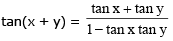now, cot x + cot y = 49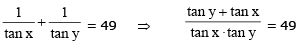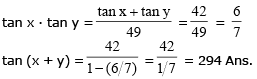Example 5. If x sinθ = y sin(θ + 2π/3) = z sin (θ + 4π/3) then:
(a) x + y + z = 0
(b) xy + yz + zx = 0
(c) xyz + x + y + z = 1
(d) none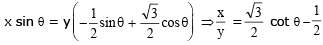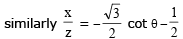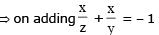= xy + yz + zx = 0

Example 6. Find θ satisfying the equation, tan 15° . tan 25° . tan 35° = tan 0, where θ ∈ (0, 15°).

LHS = tan 15° . tan (30° - 5°) . tan (30° + 5°)
let t = tan 30° and m = tan 5°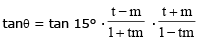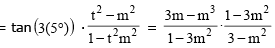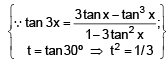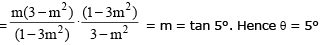Example 7. If tan A & tan B are the roots of the quadratic equation, a x2 + b x + c = 0 then evaluate a sin2 (A + B) + b sin (A + B) . cos (A + B) + c cos2 (A + B).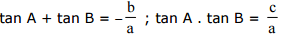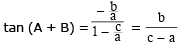Now E = cos2 (A + B) [a tan2 (A + B) + b tan (A + B) + c]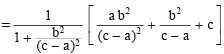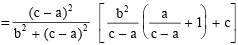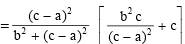Example 8. Show that cos2A + cos2(A + B) + 2 cosA cos(180° + B) · cos(360° + A + B) is independent of A. Hence find its value when B = 810°.

cos2A + cos2(A + B) - [2 cosA · cosB · cos (A + B)]
= cos2A + cos2(A + B) - [ {cos(A + B) + cos(A - B) } cos (A + B) ]
= cos2A + cos2(A + B) - cos2(A + B) - (cos2A - sin2B)
= sin2B which is independent of A
now,  sin2(810°) = sin2(720° + 90°) = sin290° = 1

Multiple Angles & Sub-Multiple Angles

• sin 2A = 2 sinA cosA ; sin θ = 2 Sin(θ/2)Cos(θ/2)
• cos 2A = cos²A - sin²A = 2cos²A - 1 = 1 - 2 sin²A ;
cos θ= cos² θ/2 - sin² θ/2 = 2cos² θ/2 - 1 = 1 - 2sin² θ/2.
2 cos²A = 1 + cos 2A , 2sin²A = 1 - cos 2A
2 cos² θ/2 = 1 + cos θ, 2 sin² θ/2 = 1 - cos θ.
•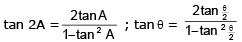•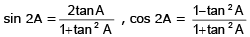• cos 3A = 4 cos3A - 3 cosA
• sin 3A = 3 sinA - 4 sin3A
•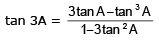Important Trigonometric Ratios

1.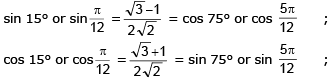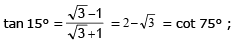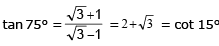2.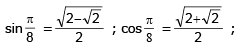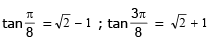3.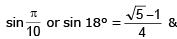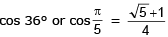Conditional Identities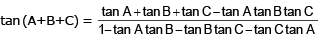If A+B+C = π

• tanA + tanB + tanC = tanA tanB tanC
•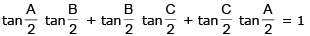• sin2A + sin2B + sin2C = 4 sinA sinB sinC
• sinA + sinB + sinC = 4 cos A/2 Cos B/2 Cos C/2
• cos 2A + cos 2B + cos 2C = –1 – 4 cos A cos B cos C
• cos A + cos B + cos C = 1 + 4 sin A/2 sin B/2 sin C/2

Example 10. If A + B + C = π, prove that,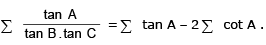Solution: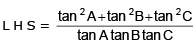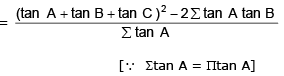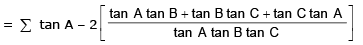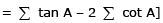Example 11. If A + B + C = θ and cotθ = cot A + cot B + cot C, show that , sin(A-θ). sin (B-θ).sin (C-θ)= sin3 θ.

Solution:

Given cot θ = cot A + cot B + cot C or cot θ - cot A = cot B + cot C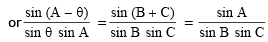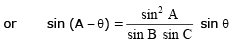....(1)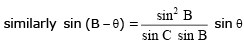....(2)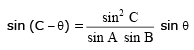....(3)

Multiplying (1) , (2) and (3) we get the result

Example 12. Find whether a triangle ABC can exists with the tangents of its interior angle satisfying, tan A = x, tan B = x + 1 and tan C = 1 - x for some real value of x. Justify your assertion with adequate reasoning.

Solution:

In a triangle ∑ tan A = π tan A (to be proved)
x + x + 1 + 1 – x = x(1 + x)(1 – x)
2 + x = x – x3; x3 = –2, x = -21/3
Hence tanA = x < 0 and tanB = x + 1 = 1 – 21/3 < 0
Hence A and B both are obtuse. Which is not possible in a triangle. Hence no such triangle can exist.

Maximum & Minimum values of Trigonometric Functions

• Min. value of a2 tan2 θ + b2 cot2θ = 2ab
• Max and Min. value of acosθ + bsinθ are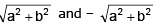• If f(θ) = acos(α + q) + bcos(β + q) where a, b, α and β are known quantities then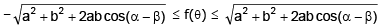•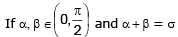(constant) then the maximum values of the expression cosα cosβ, cosα+ cosβ, sinα + sinβ and sina sinb occurs when α = β = σ/2
• If A, B, C are the anlges of a triangle then maximum value of sinA + sinB + sinC and sinA sinB sinC occurs when A = B = C = 60º
• In case a quadratic in sinq or cosq is given then the maximum or minimum values can be interpreted by making a perfect square

Example 13. Find the greatest value of c such that system of equations x2 + y2 = 25; x + y = c has a real solution.

Solution:

Put x = 5 cosθ and y = 5 sinθ

5(cosθ+ sinθ) = c; but (cosθ + sinθ)max = √2 and (cosθ + sinθ)min = – √2

hence, cmax = 5√2

Example 14. Find the minimum and maximum value of f (x, y) = 7x2 + 4xy + 3y2 subjected to x2 + y2 = 1.

Solution:

Let x = cosθ and y = sinθ
y = f (θ) = 7 cos2θ + 4 sin θcosθ + 3 sin2θ = 3 + 2 sin 2q + 2(1 + cos 2θ)
= 5 + 2(sin 2θ + cos 2θ) but -√2 ≤ (sin 2θ + cos 2θ) ≤ √2
ymax = 5 + 2√2 and ymin = 5 – 2√2

The document Important Formulas & Examples: Trigonometric Functions - 1 | Mathematics (Maths) Class 11 - Commerce is a part of the Commerce Course Mathematics (Maths) Class 11.
All you need of Commerce at this link: Commerce
FAQs on Important Formulas & Examples: Trigonometric Functions - 1 | Mathematics (Maths) Class 11 - Commerce
 1. What are the basic trigonometric identities?Ans. The basic trigonometric identities include: $\sin^2\theta+\cos^2\theta=1$, $\tan\theta=\frac{\sin\theta}{\cos\theta}$, $\cot\theta=\frac{\cos\theta}{\sin\theta}$, and $\sec\theta=\frac{1}{\cos\theta}$ and $\csc\theta=\frac{1}{\sin\theta}$.
 2. What are the important trigonometric ratios?Ans. The important trigonometric ratios include: sine ratio, cosine ratio, tangent ratio, cotangent ratio, secant ratio, and cosecant ratio.
 3. What are the trigonometric functions of sum or difference of two angles?Ans. The trigonometric functions of sum or difference of two angles include: $\sin(A \pm B)=\sin A\cos B\pm\cos A\sin B$, $\cos(A \pm B)=\cos A\cos B\mp\sin A\sin B$, and $\tan(A \pm B)=\frac{\tan A\pm\tan B}{1\mp\tan A\tan B}$.
 4. What are the maximum and minimum values of trigonometric functions?Ans. The maximum value of sine and cosine functions is 1, and the minimum value is -1. The maximum value of tangent and cotangent functions is undefined, and the minimum value is -∞ to +∞. The maximum value of secant and cosecant functions is -1 and 1, respectively, and the minimum value is -∞ to +∞.
 5. What are the conditional identities in trigonometry?Ans. The conditional identities in trigonometry include: $\sin(-\theta)=-\sin\theta$, $\cos(-\theta)=\cos\theta$, $\tan(-\theta)=-\tan\theta$, $\sin(\pi-\theta)=\sin\theta$, $\cos(\pi-\theta)=-\cos\theta$, and $\tan(\pi-\theta)=-\tan\theta$.

## Mathematics (Maths) Class 11

156 videos|176 docs|132 tests

## Mathematics (Maths) Class 11

156 videos|176 docs|132 tests

Track your progress, build streaks, highlight & save important lessons and more!(Scan QR code)

,

,

,

,

,

,

,

,

,

,

,

,

,

,

,

,

,

,

,

,

,

;